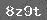# 行走在教什么和怎么教之间

【片段回放】笔者校本教研研讨课《加法交换律》

 5+8=1331+26=57306+139=4457189+811=800050780+13658=64438 8+5=26+31=139+306=811+7189=13658+50780=

……

【片段回放】笔者参加市教坛新秀评比考核课《三角形的认识》

（生摆三角形，但没有办法摆出不一样的三角形）

……

……

【片段回放】笔者校本教研展示课《分数的意义》

 范新林.让学生从明了形式走向领悟本质[J].教学月刊，2011(1-2)：4-5。

 唐彩斌.关于小学“数学本质”的对话[J].人民教育，2009(2)。

#  李昕阳.从概率计算看数学本质教学[J].中学数学杂志(初中版)，2009(4)。

### 相关推荐

 留言与评论（共有 0 条评论）

 验证码：